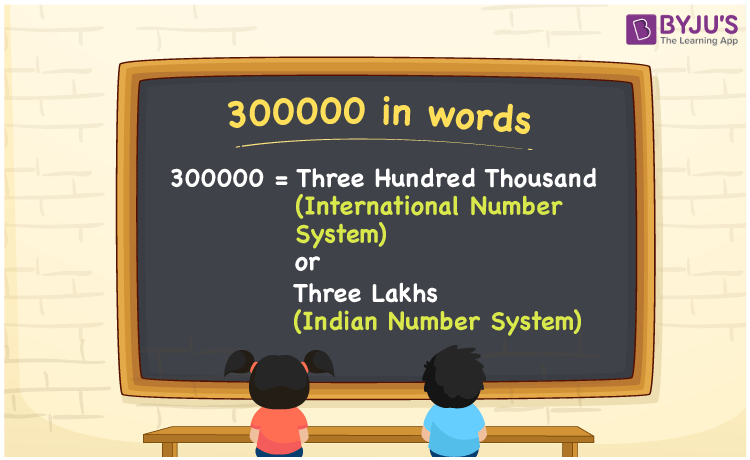# 300000 in Words

300000 in words is written as Three Hundred Thousand in the International System of Numerals. In the Indian System of Numerals, 300000 is written as three lakhs. The number 300000 is a Cardinal Number as it could represent some quantity. For example, “Frank has 300000 rupees in his bank account”.

 300000 International System of Numerals Indian System of Numerals In Words Three Hundred Thousand Three Lakhs In Number 300,000 3,00,000

## 300000 in English Words

We write 300000 in English Words using the letters of the English alphabet. Therefore, we read 300000 in English as “Three Hundred Thousand” or “Three lakhs.”## How to Write 300000 in Words?

To write 300000 in words, we shall use the place value chart. In the place value chart, write 3 in the hundred thousand place and 0 in the rest. Let us make a place value chart to write the number 300000 in words.

In the International System of Numerals,

 Hundred Thousand Ten Thousands Thousands Hundreds Tens Ones 3 0 0 0 0 0

In the Indian System of Numerals.

 Lakhs Ten Thousands Thousands Hundreds Tens Ones 3 0 0 0 0 0

Thus, we can write the expanded form as

3 × Hundred Thousands + 0 × Ten Thousand + 0 × Thousand + 0 × Hundred + 0 × Ten + 0 × One

= 3 × 100,000 + 0 × 10,000 + 0 × 1000 + 0 × 100 + 0 × 10 + 0 × 1

= 300,000 + 0 + 0 + 0 + 0 + 0

= 300,000

= Three Hundred Thousand

3 × Lakh + 0 × Ten Thousand + 0 × Thousand + 0 × Hundred + 0 × Ten + 0 × One

= 3 × 1,00,000 + 0 × 10,000 + 0 × 1000 + 0 × 100 + 0 × 10 + 0 × 1

= 3,00,000 + 0 + 0 + 0 + 0 + 0

= 3,00,000

= Three lakhs.

300000 is a natural number that lies between 299999 and 300001.

300000 in words – Three Hundred Thousand or Three lakhs

• Is 300000 an odd number? – No
• Is 300000 an even number? – Yes
• Is 300000 a perfect square number? – No
• Is 300000 a perfect cube number? – No
• Is 300000 a prime number? – No
• Is 300000 a composite number? – Yes

## Frequently Asked Questions on 300000 in Words

Q1

### How to write 300000 in words?

300000 in words is written as Three Hundred Thousand.
Q2

### How to write 300000 in the International and Indian System of Numerals?

In International System, 300000 is written as 300,000 and read as three hundred thousand. In Indian System, 300000 is written as 3,00,000 and read as three lakhs.
Q3

### What is the successor of 300000?

The successor of 300000 is 300001.